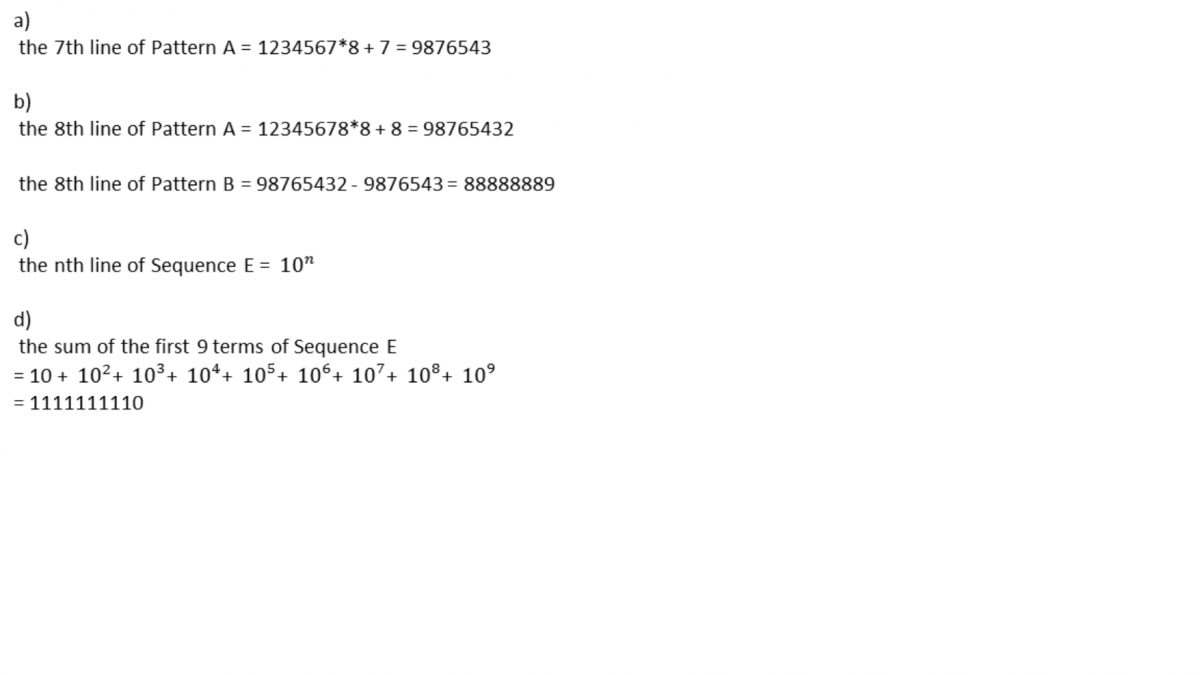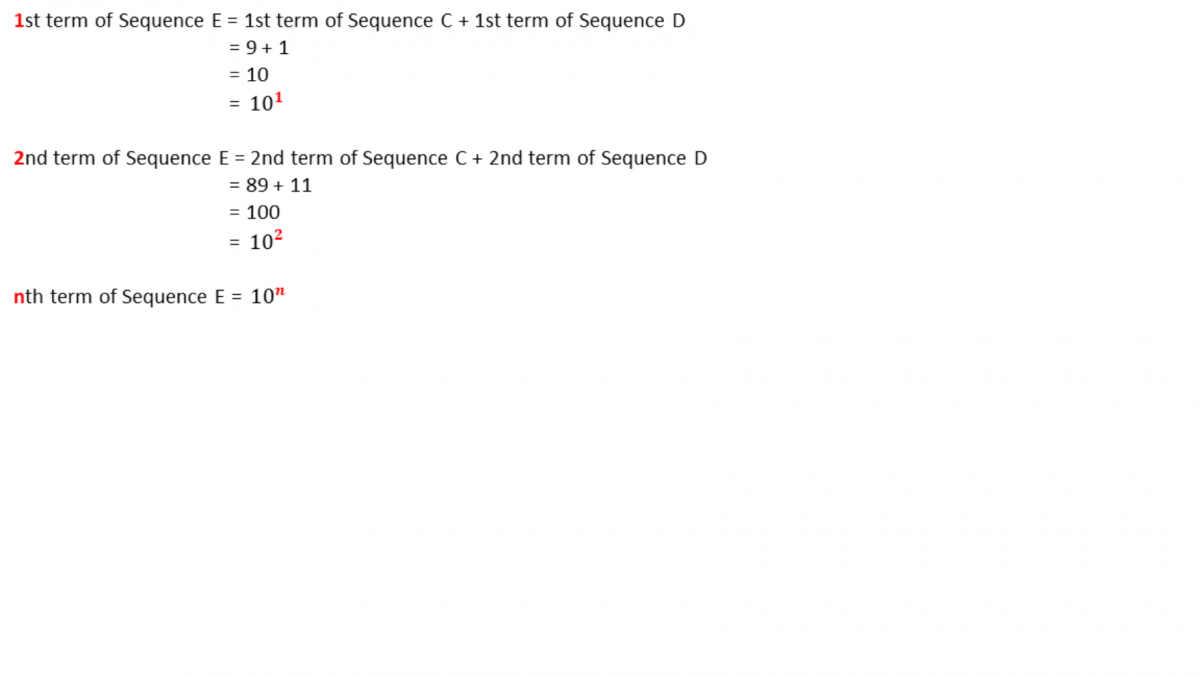# Question

Hi all, ps help. Many this and God Bless:)

Observe the patterns:

Pattern A           Pattern B

1×8+1=9             9-0=9

12×8+2=98        98-9=89

123×8+3=987    987-98=889

(a) Write down the 7th line of the Pattern A

(b) Deduce the 8th line of the Pattern B

Sequence C is given as:  9, 89, 889, ….

Sequence D is given as:  1, 11, 111, ….

Sequence E has the following rule

nth term of Sequence E = nth term of Sequence C + nth term of Sequence D

(c) Write the nth term of Sequence E as a power of 10

(d) Hence find the sum of the first 9 terms of Sequene E.